Quantity: 0

Total: 0,00

0

# Volume of spheres (Cavalieri´s principle)

### Volume of spheres (Cavalieri´s principle)

Calculating the volume of a sphere is possible using an appropriate cylinder and cone.

Mathematics

Keywords

volume of sphere, Cavalieri´s principle, calculating volume, solids, sphere, mathematics

Related items

### Scenes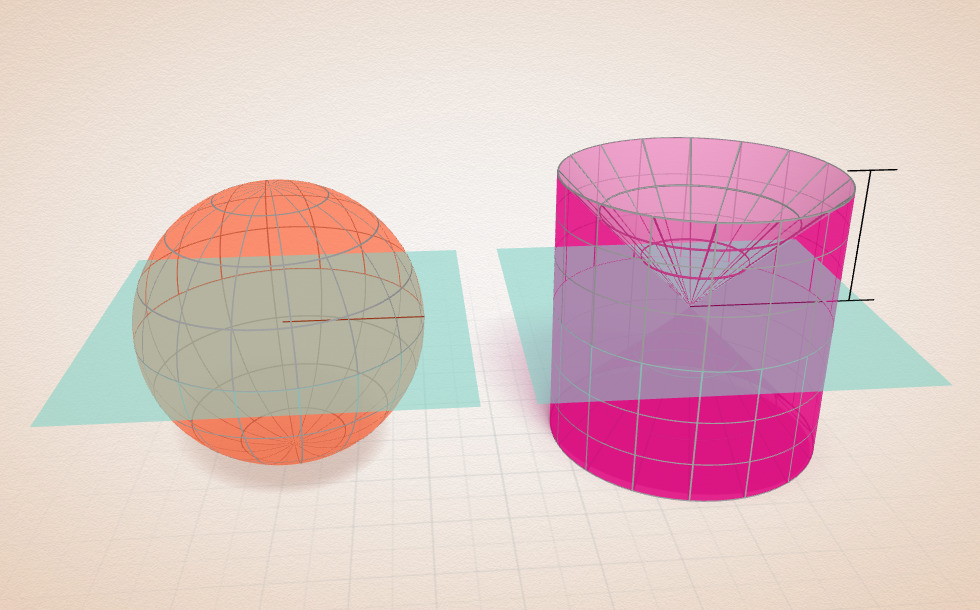• r

### Cavalieri´s principle

Take two solids and place them on one plane. Intersect them with two planes parallel to their base and examine the two solids and their cross-sections according to the following properties:
- The areas of their bases are equal.
- The areas of all cross sections parallel to their bases are equal.
- The heights of the two solids are equal.

If all of these are true, the volumes of the two solids are equal.

Cavalieri´s principle helps a lot in calculating the volume of spheres. Without it, higher mathematical methods would need to be applied in order to come to a result.

Let´s consider a hemisphere of radius r with its cross-section, and a cylinder which lies in the same plane. The radius of the circular base and the height of the cylinder are r. Let´s cut from the cylinder an upturned cone, with both radius and height r. In the animation, these solids are shown together with their mirror images relative to the plane. The areas of the bases of the two solids are equal.

When examining their cross-sections parallel to this plane we have to calculate the area of the cross-sections, which is at a height h.

In the case of the sphere the cross-section is a circle. Due to the Pythagorean theorem, the square of the circle's radius equals to r² - h² , thus its area isIn the case of the other solid the cross-section is an annulus with an external radius r and an internal radius h. Its area isIn other words, given two solids, the surface areas of their cross-sections parallel to their base are equal.
Due to the formation of the two solids, the heights of the two solids are equal.

All the conditions of Cavalieri´s principle are met, therefore the two solids have an equal volume.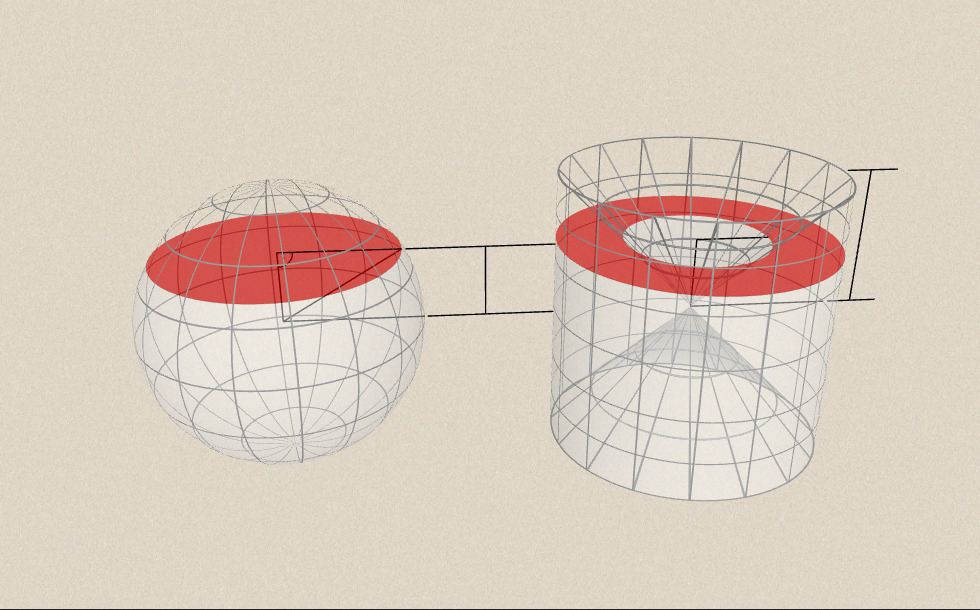• h

### Related items#### Sphere

A sphere is the set of points which are all within the same distance from a given point in space.#### Volume of spheres (demonstration)

The sum of the volume of the ´tetrahedrons´ gives an approximation of the volume of the sphere.#### Perimeter, area, surface area and volume

This animation presents the formulas to calculate the perimeter and area of shapes as well as the surface area and volume of solids.#### Physicists who changed the world

These great scientists had a tremendous impact on the advancement in physics.#### Ratio of volumes of similar solids

This 3D scene explains the correlation between the ratio of similarity and the ratio of volume of geometric solids.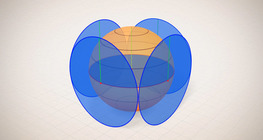#### Surface area of spheres (demonstration)

The surface of a sphere consists of the set of points which are all at the same distance from a given point in space.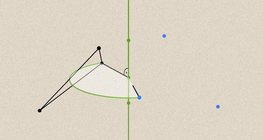#### Geometric transformations – rotation

This animation demonstrates geometric rotation, a type of geometric transformation both in plane and space.#### Solids of revolution

Rotating a geometric shape around a line within its geometric plane as an axis results in a solid of revolution.#### Volume and surface area (exercise)

An exercise about the volume and surface area of solids generated from a ´base cube´.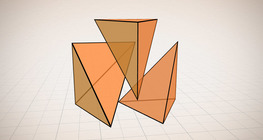#### Volume of a tetrahedron

To calculate the volume of a tetrahedron we start by calculating the volume of a prism.